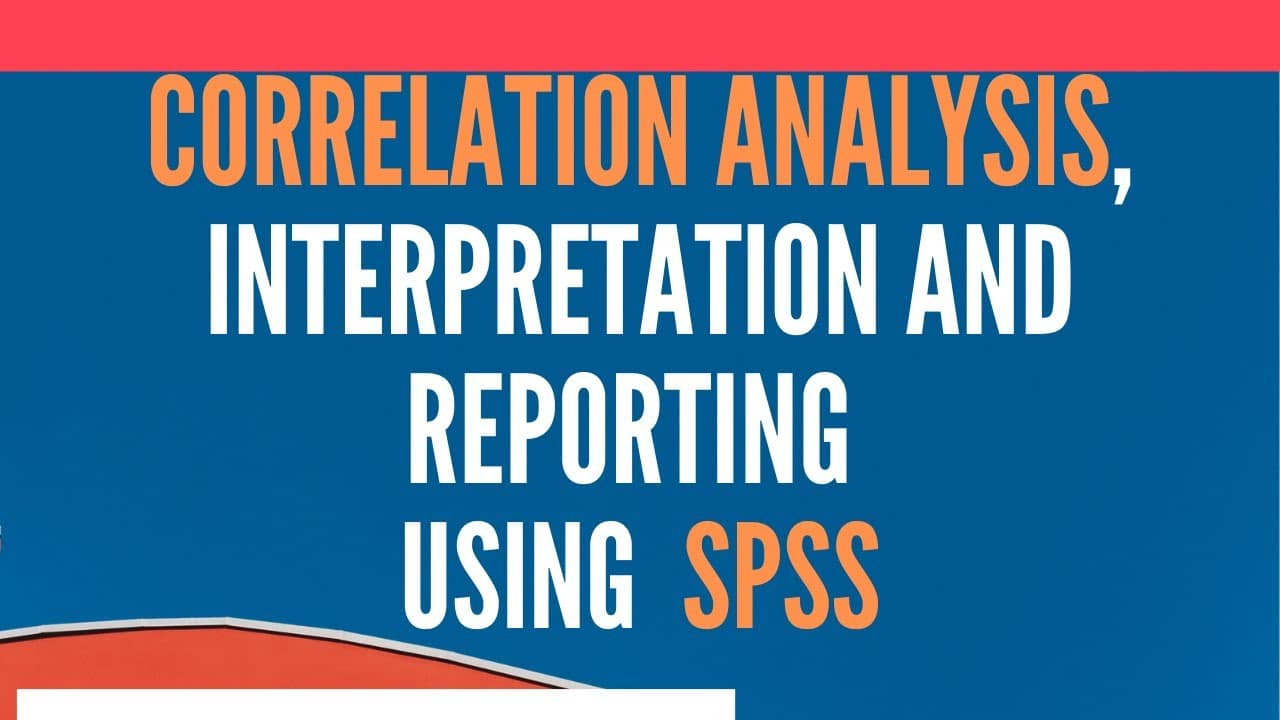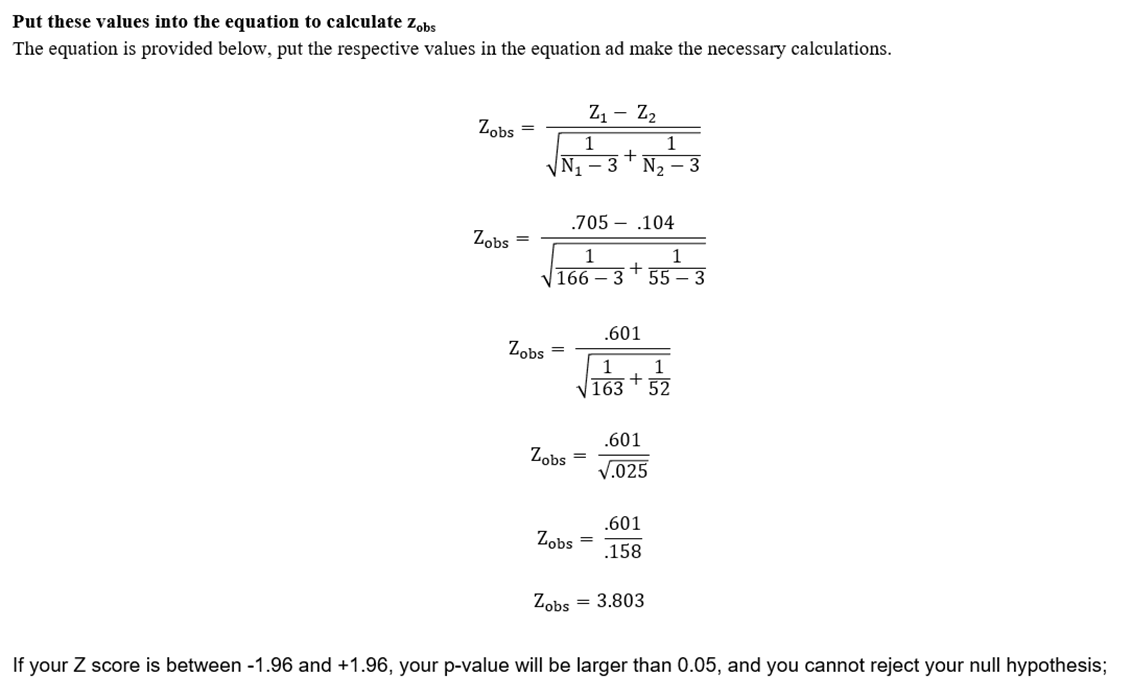# Pearson Correlation Analysis using SPSS#### Pearson Correlation Analysis using SPSS

The tutorial guides the researchers on the concept, interpretation, and reporting Pearson Correlation Analysis using SPSS.

Tutorials include

1. Concept of Correlation Analysis.
2. Pearson Correlation and Its Interpretation.
3. Correlation Matrix
4. Reporting Correlation Results

### What is Correlation?

Correlation is a measure of relationship between two variables. It has wide application in business and statistics. Now what would you do when you have two variables that are needed to be studied together. How would you use SPSS to identify relationship, association, link or bonding of two variables at one time. The analysis of relationship between two variables is termed as correlation analysis.   Correlation analysis is used to describe the strength and direction of the linear relationship between two variables.

### Example Scenarios

Following are few scenarios in which we would go for Correlation Analysis

• A university would like to know if social responsibility is associated with university reputation in the community
• A marketing manager would like to know if a hike in prices has anything to do with decrease in product sales
• A HR manager would like to know if an increase in employee pay would result in lower absenteeism
• A social science researcher would like to know if increase in age results in decrease of conflicts at work/home

### Pearson Correlation

Pearson r is designed for interval/ratio level (continuous) variables. It can also be used if you have one continuous variable (Employee Commitment) and one dichotomous variable (e.g. Type of Organization: Public/Private). The correlation is expressed in the form of a coefficient. Symbolized as r, a correlation coefficient is normally reported as a decimal number somewhere between +1.00 and -1.00.

#### Direction of Relationship

Positive, Negative or Zero Correlation

• The positive and negative signs indicate positive and negative correlation respectively. Positive sign shows that with increase in one variable, the other increases as well, while negative correlation indicates that with an increase in one variable, the other decreases. However, the value itself provides an indication of the strength of relationship.
• If the correlation is 1 or –1, this shows perfect correlation. A perfect correlation shows that the value of one variable can be completely determined by the other variable.
• If the correlation coefficient is 0, this shows there is no relationship between the two variables. Hence, one variable provides no support in predicting the second variable.

#### Interpretation of the Correlation Coefficient

It is important that we know what is meant by the coefficient, what it tells about the data. To have an answer, generally, the coefficient of correlation is interpreted in verbal description. Correlation Coefficient can easily be interpreted using the following table.

 Size of Correlation Interpretation 1 Perfect Positive/Negative Correlation ± .90 to  ± .99 Very High Positive/Negative Correlation ± .70 to ± .90 High Positive/Negative Correlation ± .50 to ± .70 Moderate Positive/Negative Correlation ± .30 to ± .50 Low Positive/Negative Correlation ± .10 to ± .30 Very low Positive/Negative Correlation ± .0 to ± .10 Markedly Low and Negligible Positive/Negative Correlation

It is important to note that correlation does not provide any information about cause and effect. The correlation does not speak of the influence one variable has over the other. It is important that you remember that correlation is not equal to causation.

#### Correlation is not Cause and Effect Analysis

It is important to note that correlation does not provide any information about cause and effect. The correlation does not speak of the influence one variable has over the other. It is important that you remember that correlation is not equal to causation.

#### Assumptions of Pearson Correlation

• The two variables shall be on Interval or Ratio scale or
• One variable can be continuous whereas the other can be binary (dichotomous).
• The continuous variable in the person correlation analysis shall be normally distributed.

#### Pearson Correlation Example

The Problem:

Investigate the relationship between Servant Leadership (SL) and Self Efficacy (SE).

Hypothesis

HA: There is a significant relationship between Servant Leadership and Self Efficacy.

Information Required:

• Two continuous variables (In this case, Servant Leadership and Self Efficacy.

### Pearson Correlation Analysis using SPSS - Steps

1. Go to Analyze → Correlate → Bivariate
2. Select the variables for which the correlation is to be studied from the list box on the left  and press the arrow to move them to the list box on the right side. Correlation requires at least two variables in the box on the right.
3. Select the variables, Press the Arrow button to the add the variable to the Variables list box
4. Make sure you have selected Pearson Coefficient checkbox from the Correlation Coefficient group box.

#### Choosing the Options

1. Next, while choosing between one-tailed and two-tailed test of significance, One-tailed test is select if the direction of relationship (Positive or Negative is known) otherwise if the direction of relationship is unknown select two-tailed test.
2. Finally Flag significant correlations to show an asterisk next to each correlation that is significant at the 0.05 significance level and two asterisks next to each correlation that is significant at the 0.01 significance level.
3. Press OK

#### Correlation Matrix

• Correlation is often used to explore the relationship among more than two variables. To do so, add more than two variables in the variables list box while selecting the variables form the list of variables.

### Report Pearson Correlation Analysis using SPSS

The Problem:

Investigate the relationship between servant leadership and self-efficacy.

H1: There is significant relationship between servant leadership and self-efficacy.

Pearson product correlation of servant leadership and self-efficacy was found to be moderately positive and statistically significant (r = .534, p < .001). Hence, H1 was supported. This shows that an increase in servant leadership behavior would lead to a higher self-efficacy in the followers.

### Groupwise Correlation Analysis

There may be situations when you need to compare the correlation coefficient between two groups. For instance, we may need to compare how the correlation between two variables differs between male and female respondents.

Statistical Significance for the difference between Groups

• While you now know how to find a correlation coefficient in each of the groups, but still we do not know if the difference in the relationship between groups is significant. Unfortunately, SPSS will not do this step for you, so it is done manually.
• First, we will be converting the r values into z scores and then we use an equation to calculate the observed value of Z. The value obtained will be assessed using a set decision rule to determine the likelihood that the difference in the correlation noted between the two groups could have been due to chance.

#### Groupwise Correlation - Convert each of the r values into z values

• The first step is to convert the correlation coefficients (r) into the Z scores. From the SPSS output, find the r-value (ignore any negative sign out the front) and N for Group 1 (males) and Group 2 (females).

To calculate the Z score, we can use the Fisher Z transformation. Open Excel and type the formula (= Fisher(Correlation Coefficient to be Converted)

• Males ( r ) = .608 n = 166
• Females ( r ) = .104 n = 55
• Males z = .705
• Female z = .104